# Natural number

In mathematics, the natural numbers are those numbers used for counting (as in "there are six coins on the table") and ordering (as in "this is the third largest city in the country"). Numbers used for counting are called cardinal numbers, and numbers used for ordering are called ordinal numbers. Natural numbers are sometimes used as labels, known as nominal numbers, having none of the properties of numbers in a mathematical sense (e.g. sports jersey numbers). Some definitions, including the standard ISO 80000-2, begin the natural numbers with 0, corresponding to the non-negative integers 0, 1, 2, 3, ..., whereas others start with 1, corresponding to the positive integers 1, 2, 3, ... Texts that exclude zero from the natural numbers sometimes refer to the natural numbers together with zero as the whole numbers, while in other writings, that term is used instead for the integers (including negative integers). The natural numbers form a set. Many other number sets are built by successively extending the set of natural numbers: the integers, by including an additive identity 0 (if not yet in) and an additive inverse −n for each nonzero natural number n; the rational numbers, by including a multiplicative inverse for each nonzero integer n (and also the product of these inverses by integers); the real numbers by including the limits of (converging) Cauchy sequences of rationals; the complex numbers, by adjoining to the real numbers a square root of −1 (and also the sums and products thereof); and so on. This chain of extensions canonically embeds the natural numbers in the other number systems. Properties of the natural numbers, such as divisibility and the distribution of prime numbers, are studied in number theory. Problems concerning counting and ordering, such as partitioning and enumerations, are studied in combinatorics. In common language, particularly in primary school education, natural numbers may be called counting numbers to intuitively exclude the negative integers and zero, and also to contrast the discreteness of counting to the continuity of measurement—a hallmark characteristic of real numbers. (Wikipedia).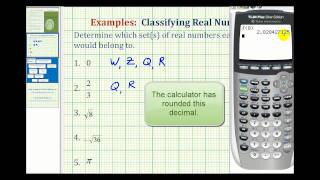Identifying Sets of Real Numbers

This video provides several examples of identifying the sets a real number belongs to. Complete Video Library: http://www.mathispower4u.com Search by Topic: http://www.mathispower4u.wordpress.com

From playlist Number Sense - Properties of Real Numbers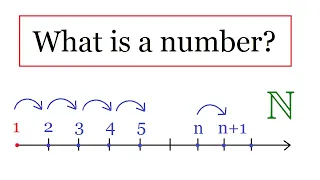What is a number ?

Construction of Natural Numbers In this, I rigorously define the concept of a natural number, using Peano's axioms. I also explain why those axioms are the basis for the principle of mathematical induction. Enjoy! Check out my Real Numbers Playlist: https://www.youtube.com/playlist?list=

From playlist Real Numbers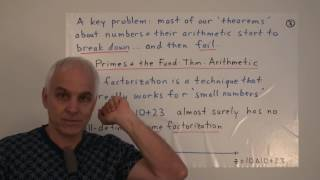Reconsidering natural numbers and arithmetical expressions | Data structures Math Foundations 185

It is time to turn our gaze back to the true foundations of the subject: arithmetic with natural numbers. But now we know that the issue of "What exactly is a natural number?" is fraught with subtlety. We adopt a famous dictum of Errett Bishop, and start to make meaningful distinctions bet

From playlist Math Foundations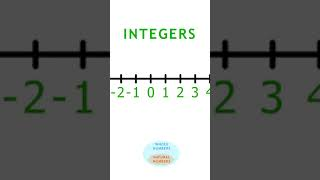Different Types of Numbers on the number line, lesson 1 #shorts

Watch the full playlist: https://www.youtube.com/watch?v=kcxK3_sROZA&list=PL14bv5vXK2WWuODhGbpPQA0GamV5ohOVb&index=1 Natural Numbers (N), (also called positive integers, counting numbers, or natural numbers); They are the numbers {1, 2, 3, 4, 5, …} Whole Numbers (W). This is the set of na

From playlist Celebrities Teach Math: The Number System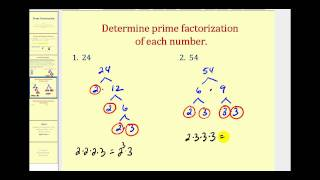Prime Factorization

This video explains how to determine the prime factorization of a number using a factor tree. http://mathispower4u.yolasite.com/

From playlist Number Sense - Whole Numbers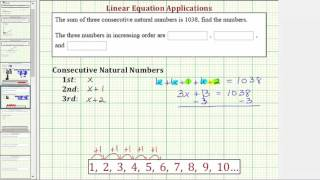Ex: Write and Solve an Equation for Consecutive Natural Numbers with a Given Sum

This video explains how to set up and solve an equation involving consecutive natural numbers with a given sum. http://mathispower4u.com

From playlist Applications: Writing and Solving Equations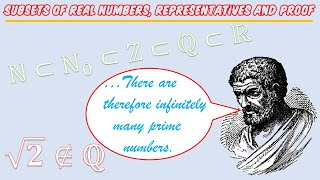Year 13/A2 Pure Chapter 0.1 (Subsets of Real Numbers, Representatives and Proof)

Welcome to the first video for year 13 (A2) Pure Mathematics! This video is part of a series of three that I've called Chapter 0, and is meant as a foundation for Year 13. The primary reasons for doing this are that the difficulty of Year 13 is markedly harder than Year 12 content, and al

From playlist Year 13/A2 Pure MathematicsSet Theory (Part 7): Natural Numbers and Induction

Please feel free to leave comments/questions on the video and practice problems below! In this video, I discuss the von Neumann construction of the natural numbers and relate the idea of natural numbers to inductive sets. The axiom of infinity is also introduced here as one of the ZFC axi

From playlist Set Theory by Mathoma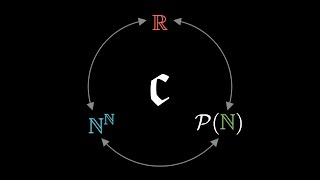Cardinality of the Continuum

What is infinity? Can there be different sizes of infinity? Surprisingly, the answer is yes. In fact, there are many different ways to make bigger infinite sets. In this video, a few different sets of infinities will be explored, including their surprising differences and even more surpris

From playlist Summer of Math Exposition 2 videos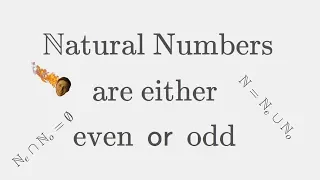Natural Numbers can be either Even OR Odd - 2 Proofs & Partition of the Positive Integers

Merch :v - https://teespring.com/de/stores/papaflammy Help me create more free content! =) https://www.patreon.com/mathable Set Theory: https://www.youtube.com/watch?v=nvYqkhZFzyY Good mornin my sons and daugthers! Let us perform anice litle task today: Showing the video titles theorem

From playlist Number TheoryReal Analysis Lecture 1.1 The Natural Numbers

00:00 Start 00:11 Overview 01:38 Natural Numbers 08:00 A Joke 12:02 Tiling By Trominos 33:41 Integers Full Playlist: https://www.youtube.com/playlist?list=PLX2fVLMrzfneCYOpe6UrBhhFDo3JNglke Suggestion: Play at 1.25 times the normal speed. Note: The auto-generated subtitles are mostly acc

From playlist Summer of Math Exposition Youtube Videos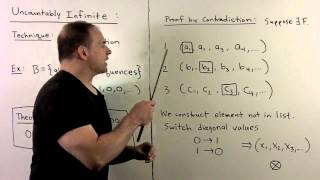BM9.2. Cardinality 2: Infinite Sets

Basic Methods: We continue the study of cardinality with infinite sets. First the class of countably infinite sets is considered, and basic results given. Then we give examples of uncountable sets using Cantor diagonalization arguments.

From playlist Math Major Basics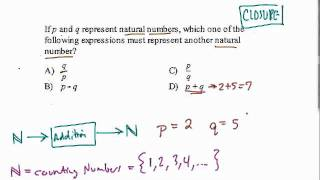Closure and Natural Numbers

Understanding the basic principles of closure for natural numbers

From playlist Geometry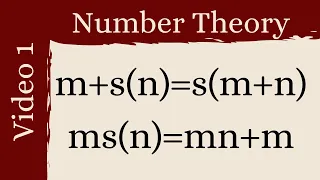The foundation -- Number Theory 1

Suggest a problem: https://forms.gle/ea7Pw7HcKePGB4my5 Please Subscribe: https://www.youtube.com/michaelpennmath?sub_confirmation=1 Merch: https://teespring.com/stores/michael-penn-math Personal Website: http://www.michael-penn.net Randolph College Math: http://www.randolphcollege.edu/ma

From playlist Number Theory v2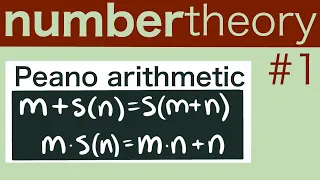Peano Arithmetic -- Number Theory 1

⭐Support the channel⭐ Patreon: https://www.patreon.com/michaelpennmath Merch: https://teespring.com/stores/michael-penn-math My amazon shop: https://www.amazon.com/shop/michaelpenn ⭐my other channels⭐ Main Channel: https://www.youtube.com/michaelpennmath non-math podcast: http

From playlist Number Theory